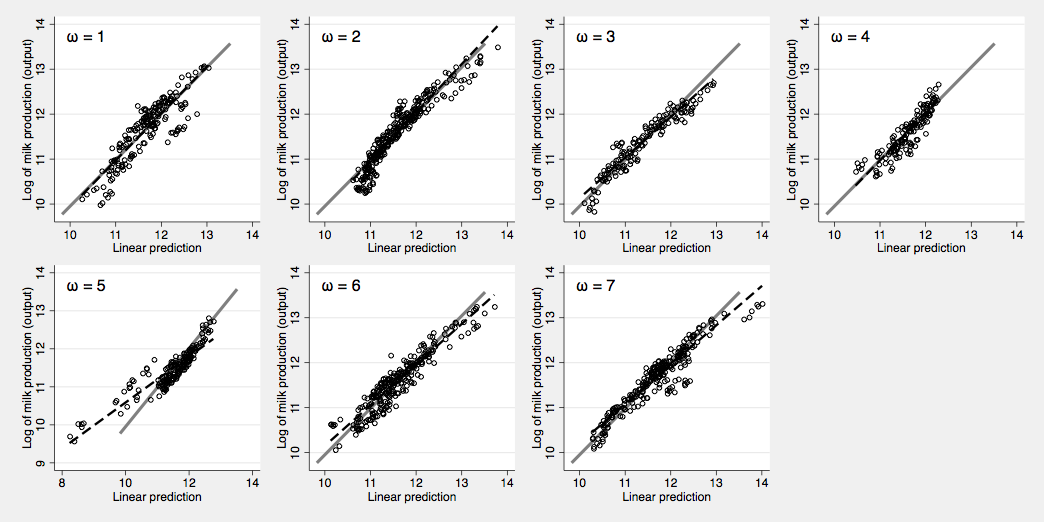# Stata-Python交互-7：在Stata中实现机器学习-支持向量机

Stata连享会   主页 || 视频 || 推文 || 知乎

New！ `lianxh` 命令发布了：

`. ssc install lianxh`

`. help lianxh`

E-Maillvzy20@163.com

Source： Chuck Huber, 2020, Stata/Python integration part 7: Machine learning with support vector machines, -Link-

Stata/Python 交互系列推文 源自 Stata 公司的统计项目总监 Chuck Huber 博士发表于 Stata 官网的系列博文，一共 9 篇。较为系统地介绍了 Stata 与 Python 的交互方式，包括：如何配置你的软件、如何实现 Stata 与 Python 数据集互通、如何调用 Python 工具包、如何进行机器学习分析等。

• Part 1: Setting up Stata to use Python -Link-
• Part 2: Three ways to use Python in Stata -Link-
• Part 3: How to install Python packages -Link-
• Part 4: How to use Python packages -Link-
• Part 5: Three-dimensional surface plots of marginal predictions -Link-
• Part 6: Working with APIs and JSON data -Link-
• Part 7: Machine learning with support vector machines, -Link-
• Part 8: Using the Stata Function Interface to copy data from Stata to Python, -Link-
• Part 9: Using the Stata Function Interface to copy data from Python to Stata, -Link-

Stata16 已具有和 python 交互的功能，由此，我们可以在 Stata 中调用 python，也可以在 python 中读取 Stata 数据，从而实现“他山之石，可以攻玉”。本节我们将向大家介绍在 stata 中实现机器学习，我们将使用一个支持向量机（SVM）的栗子进行说明。

## 1. 数据探索性分析

``````. list in 1/5

+------------------------+
| diabetes   HbA1c   age |
|------------------------|
1. |        1       7    62 |
2. |        0     5.5    53 |
3. |        1     5.8    78 |
4. |        0     5.6    56 |
5. |        0     5.6    42 |
+------------------------+
``````

``````. tabulate diabetes

Doctor told |
you have |
diabetes |      Freq.     Percent        Cum.
------------+-----------------------------------
0 |      5,531       87.49       87.49
1 |        791       12.51      100.00
------------+-----------------------------------
Total |      6,322      100.00
``````

``````python:
# Import the necessary packages
import pandas as pd
import matplotlib.pyplot as plt
import matplotlib.colors as mcolors

# Read the Stata dataset into Python
convert_categoricals=False,
preserve_dtypes=True,
convert_missing=False)

# Define the feature matrix (independent variables)
# and the target variable (dependent variable)
X = data[['age','HbA1c']]
y = data['diabetes']

# Plot the raw data
plt.scatter(X['age'], X['HbA1c'],
c=y,
cmap = mcolors.ListedColormap(["navy", "darkred"]))
plt.xlabel('Age (years)')
plt.ylabel('HbA1c')
plt.xticks((12,20,30,40,50,60,70,80))
plt.yticks((4,6,8,10,12,14,16))
plt.title('Diabetes status by Age and HbA1c')
plt.show()
# Save the graph
plt.savefig("scatterplot.png")
end
``````由图中显示，患有糖尿病的人往往年龄较大、糖化血红蛋白的水平较高。

## 2. 使用交叉验证来拟合最优 SVM 模型

``````python:
from sklearn.model_selection import train_test_split

X_train, X_test, y_train, y_test = train_test_split(X, y, test_size=0.4,
random_state=0)
end
``````

``````python:
from sklearn import svm
from sklearn.model_selection import cross_val_score
from sklearn.model_selection import GridSearchCV

# Do a grid search for the parameters "degree" and "C" using 10-fold
# cross-validation
model = svm.SVC(kernel='poly')
parameters = {'degree':[1,2,3], 'C':[1,2,3]}
poly_svc = GridSearchCV(model,
parameters,
cv=10,
scoring='accuracy').fit(X_train, y_train)

# Display the parameters that yield the best-fitting model
poly_svc.fit(X_train,y_train)
print(poly_svc.best_params_)
end

``````

``````>>> poly_svc.fit(X_train,y_train)
GridSearchCV(cv=10, estimator=SVC(kernel='poly'),
param_grid={'C': [1, 2, 3], 'degree': [1, 2, 3]},
scoring='accuracy')
>>> print(poly_svc.best_params_)
{'C': 3, 'degree': 3}
``````

## 3. 在测试集上拟合模型

``````# Fit the SVM model using the parameters selected from the grid search
poly_svc = svm.SVC(kernel='poly', degree=3, C=3).fit(X_train, y_train)
scores = cross_val_score(poly_svc, X_test, y_test, cv=10, scoring='accuracy')
print("Accuracy: %0.2f (+/- %0.2f)" % (scores.mean(), scores.std() * 2))

>>> Accuracy: 0.93 (+/- 0.03)
``````

## 6. 相关推文

Note：产生如下推文列表的命令为：
`lianxh Stata Python +`

`ssc install lianxh, replace`

## 相关课程

http://lianxh.duanshu.com

### 课程一览

Note: 部分课程的资料，PPT 等可以前往 连享会-直播课 主页查看，下载。

#### 关于我们

• Stata连享会 由中山大学连玉君老师团队创办，定期分享实证分析经验。直播间 有很多视频课程，可以随时观看。
• 连享会-主页知乎专栏，400+ 推文，实证分析不再抓狂。
• 公众号关键词搜索/回复 功能已经上线。大家可以在公众号左下角点击键盘图标，输入简要关键词，以便快速呈现历史推文，获取工具软件和数据下载。常见关键词：`课程, 直播, 视频, 客服, 模型设定, 研究设计, stata, plus, 绘图, 编程, 面板, 论文重现, 可视化, RDD, DID, PSM, 合成控制法`

New！ `lianxh` 命令发布了：
`. ssc install lianxh`
`. help lianxh`